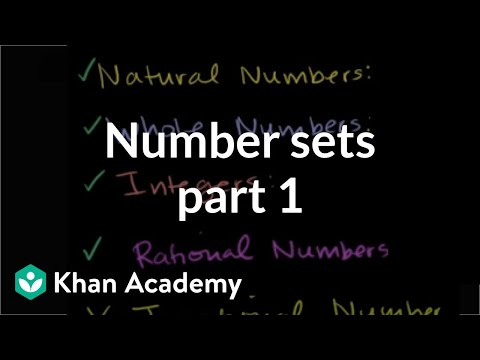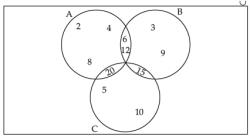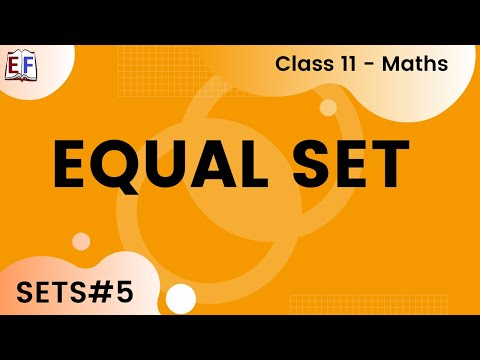Courses

# Test: Sets- 1

## 40 Questions MCQ Test Quantitative Aptitude for CA CPT | Test: Sets- 1

Description
This mock test of Test: Sets- 1 for CA Foundation helps you for every CA Foundation entrance exam. This contains 40 Multiple Choice Questions for CA Foundation Test: Sets- 1 (mcq) to study with solutions a complete question bank. The solved questions answers in this Test: Sets- 1 quiz give you a good mix of easy questions and tough questions. CA Foundation students definitely take this Test: Sets- 1 exercise for a better result in the exam. You can find other Test: Sets- 1 extra questions, long questions & short questions for CA Foundation on EduRev as well by searching above.
QUESTION: 1

### Choose the most appropriate option or options (a), (b) (c) and (d) The number of subsets of the set {2, 3, 5} is

Solution:

The subsets of set are:

{ (),(2),(3),(5),(2,3),(2,5),(3,5),(2,3,5) }
Total eight subsets.

Shortcut to find number of subsets:
2n
where n = no.of elements in a set.

Applying this shortcut in the above set,
n=3

2= 8

QUESTION: 2

Solution:
QUESTION: 3

### The null set is represented by

Solution:
QUESTION: 4

A = {2, 3, 5, 7} , B { 4, 6, 8, 10} then A ∩ B can be written as

Solution:
QUESTION: 5

The set {x|0<x<5} represents the set when x may take integral values only

Solution:
QUESTION: 6

The set {0, 2, 4, 6, 8, 10} can be written as

Solution:
QUESTION: 7

If P = {1, 2, 3, 5, 7}, Q = {1, 3, 6, 10, 15}, Universal Set S = {1, 2, 3, 4, 5, 6, 7, 8, 9, 10, 11, 12, 13, 14, 15}

Q. The cardinal number of P ∩ Q is

Solution:

Cardinal number means whole number that shows quantity
P ∩ Q = { 1,3}
P ∩ Q i.e. all elements of P that also belong to Q
Cardinal number of P ∩ Q = 2

QUESTION: 8

If P = {1, 2, 3, 5, 7}, Q = {1, 3, 6, 10, 15}, Universal Set S = {1, 2, 3, 4, 5, 6, 7, 8, 9, 10, 11, 12, 13, 14, 15}

Q. The cardinal number of P ∪ Q is

Solution:
QUESTION: 9

If P = {1, 2, 3, 5, 7}, Q = {1, 3, 6, 10, 15}, Universal Set S = {1, 2, 3, 4, 5, 6, 7, 8, 9, 10, 11, 12, 13, 14, 15}

Q. n(P1) is

Solution:
QUESTION: 10

If P = {1, 2, 3, 5, 7}, Q = {1, 3, 6, 10, 15}, Universal Set S = {1, 2, 3, 4, 5, 6, 7, 8, 9, 10, 11, 12, 13, 14, 15}

Q. n(Q1) is

Solution:
QUESTION: 11

The set of cubes of the natural number is

Solution:
QUESTION: 12

The set {2x|x is any positive rational number } is

Solution:
QUESTION: 13

{1– (–1)x} for all integral x is the set

Solution:
QUESTION: 14

E is a set of positive even number and O is a set of positive odd numbers, then E ∪ O is a

Solution:
QUESTION: 15

If R is the set of positive rational number and E is the set of real numbers then

Solution:
QUESTION: 16

If N is the set of natural numbers and I is the set of positive integers, then

Solution:
QUESTION: 17

If I is the set of isosceles triangles and E is the set of equilateral triangles, then

Solution:

Since every equilateral triangle is also an isoceles triangle then E is a subset of I.

QUESTION: 18

If R is the set of isosceles right angled triangles and I is set of isosceles triangles, then

Solution:

If R is the set of isosceles right angled triangles and l is set of isosceles triangles, then R belongs to l.

(R is a subset of l)

QUESTION: 19

{n(n+1)/2 : n is a positive integer} is

Solution:
QUESTION: 20

If A = {1, 2, 3, 4}, B = {3, 4, 5, 6}, C = {5, 6, 7, 8 } and D = { 7, 8, 9, 10 }; find A ∪ B

Solution:
QUESTION: 21

A ∪ A is equal to

Solution:
QUESTION: 22

(A ∪ B)' is equal to

Solution:
QUESTION: 23

(A ∩ B)' is equal to

Solution:
QUESTION: 24

A ∪ E is equal to (E is a superset of A)

Solution:
QUESTION: 25

A ∩ E is equal to

Solution:
QUESTION: 26

E ∪ E is equal to

Solution:
QUESTION: 27

A ∩ E' is equal to

Solution:
QUESTION: 28

If P = {1, 2, 3, 5, 7}, Q = {1, 3, 6, 10, 15}, Universal Set S = {1, 2, 3, 4, 5, 6, 7, 8, 9, 10, 11, 12, 13, 14, 15}
The cardinal number of P ∩ Q is

Solution:

P = {1, 2, 3, 5, 7}, Q = {1, 3, 6, 10, 15},
P ∩ Q = { 1, 3 }.
The cardinal number is the number of elements of a set.
So The cardinal number of P ∩ Q is 2.

QUESTION: 29

A ∩ A' is equal to

Solution:
QUESTION: 30

If E = {1, 2, 3, 4, 5, 6, 7, 8, 9}, the subset of E satisfying 5 + x > 10 is

Solution:
QUESTION: 31

If A∆ B = (A–B) ∪ (B–A) and A = {1, 2, 3, 4}, B = {3,5,7} than A∆B is

Solution:
*Multiple options can be correct
QUESTION: 32

If A = {x, y, z}, B = {p, q, r, s} Which of the relation on A.B are function.

Solution:
QUESTION: 33

{(x,y)|x+y = 5} is a

Solution:
QUESTION: 34

{( x , y)|x = 4} is a

Solution:
QUESTION: 35

{(x , y), y=x2} is

Solution:
QUESTION: 36

{(x, y)|x<y} is

Solution:
QUESTION: 37

The domain of {(1,7), (2,6)} is

Solution:
QUESTION: 38

The range of {(3,0), (2,0), (1,0), (0,0)} is

Solution:
QUESTION: 39

The domain and range of {(x,y) : Y = x2} is

Solution:
QUESTION: 40

Let the domain of x be the set {1}. Which of the following functions are equal to 1

Solution:

Track your progress, build streaks, highlight & save important lessons and more!

### Similar Content### Related tests